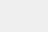# 通过JavaScript实现扑克牌游戏的示例代码

我们说下想要实现，一副牌里有54张，我们可以知道 3 – 2 的牌总共有13张，这分为4个花色是 ♠️ ♥️ ♣️ ♦️ 另外加上2个大小王！第一步：形成一个数组， 就要写一个函数，利用牌数量和花色，这样可以用来形成一个双重循环将 number 里面的内容 和 flower 里面的内容 进行一个循环嵌入？最后在用 push 生成一个对象放到数组的后面？再到最后放入 大小王 。

```　　const number = ['3', '4', '5', '6', '7', '8', '9', '10', "J", "Q", "K", "A", '2'];
const flower = ["♠️", "♥️", "♣️", "♦️"]; // 黑桃 < 红心 < 梅花 < 方块
/**
生成一个扑克牌，然后分发3副牌，1副牌中有17张牌，然后有四种颜色，底牌留三张。按照大小进行排序
* 54 张
* 大小王
* 张三： 17
* 李四： 17
* 王五： 17
* 底牌： 3
*
* 排序 方块3 黑桃3 升序 黑桃3-方块3 如果点数相同，按花色排
*/```

语法：

```　　//系统牌
const number = ['3', '4', '5', '6', '7', '8', '9', '10', "J", "Q", "K", "A", '2'];
const flower = ["♠️", "♥️", "♣️", "♦️"]; // 黑桃 < 红心 < 梅花 < 方块
//牌组
let arr = [];
//生成牌的函数
poker();
//生成一副牌
function poker() {
for (let n = 0; n < number.length; n++) {
for (let j = 0; j < flower.length; j++) {
arr.push({ name: flower[j] + number[n]})
}
}
arr.push({ name: "小王" }, { name: "大王" })
}```

输出效果图：

都知道牌要随机，那我们在进行一个打乱。可以添加一个随机生成的函数和一个去重生成下标的数组

语法：```　//系统牌
const number = ['3', '4', '5', '6', '7', '8', '9', '10', "J", "Q", "K", "A", '2'];
const flower = ["♠️", "♥️", "♣️", "♦️"]; // 黑桃 < 红心 < 梅花 < 方块
//牌组
let arr = [];
//生成牌的函数
poker();
//打乱后的拆开后的牌组
let [zhangsan, wangwu, lisi, d] = create();
//打印输出
console.log(zhangsan);
console.log(wangwu);
console.log(lisi);
console.log(d);
//生成一副牌
function poker() {
for (let n = 0; n < number.length; n++) {
for (let j = 0; j < flower.length; j++) {
arr.push({ name: flower[j] + number[n]})
}
}
arr.push({ name: "小王" }, { name: "大王" })
}
//生成一个随机数
function random(min, max) {
if (max == undefined) {
max = min;
min = 0;
}
if (min > max) {
[min, max] = [max, min];
}
let number = parseInt(Math.random() * (max - min + 1) + min)
return number;
}
//打乱
function create() {
let a = new Set();
while (a.size < 54) {
}
let arrindex = [...a];
arr = arrindex.map(item => arr[item]);
let zhangsan = arr.slice(0, 17);
let wangwu = arr.slice(17, 34);
let lisi = arr.slice(34, 51);
let d = arr.slice(51);
return [zhangsan, wangwu, lisi, d];
}```

详解：可以随机54张牌，这里就是不重复的下标需要用到 Set 来晒重，但我们知道需要随机机数的时候需要主要辨别。那就要用到map map的用意：通过指定函数处理数组（遍历数组）的每个元素，并操作数组的元素或者下标，并返回处理后的数组。 下标，我们可以用 arr 里面的元素进行找到，然后并将进行返回出来，然后再使用 slice slice的用意：用于把数组中的字符串元素转换成数字数组，元素是通过指定的分隔符进行分隔的。进行一个返回接收 我们知道 zhangsan、wangwu、lisi 是有 17 张牌的 而底牌只有三张可以得到手牌。并将函数里面的内容返回出去进行一个接收输出。

输出效果图：在到最后我们需要一个排序。当 3 和 3 比肯定是没有办法比的是不是？我们首先会对牌定义花色，再去对比数组。那我们要写一个函数进行一个排序。排序需要用到 sort sort的用意：对数组的元素进行排序，不是真实按照数字排列去排序的，按照字符进行一个比较。当需要比较数字需要重写。例如sort((a,b)=> a-b)升序 sort((a,b)=> b-a) 降序！ 在生成牌组里面需要添加 花色的大小 和 数字 的大小 ，最后进行一些列的优化代码如下：

语法：

```　　const number = ['3', '4', '5', '6', '7', '8', '9', '10', "J", "Q", "K", "A", '2'];
const flower = ["♠️", "♥️", "♣️", "♦️"]; // 黑桃 < 红心 < 梅花 < 方块
let arr = [];
poker();
let [zhangsan, wangwu, lisi, d] = create();
sort(zhangsan);
sort(wangwu);
sort(lisi);
sort(d);
console.log("张三：",zhangsan);
console.log("王五：",wangwu);
console.log("王五：",lisi);
console.log("底牌：",d);
//排序
function sort(arr) {
arr.sort((a,b)=>{if (a.number == b.number) {</p>
<p>
return a.flower - b.flower;</p>
<p>
}else{
return a.number - b.number;
}})
}
//生成一个随机数
function random(min, max) {
if (max == undefined) {
max = min;
min = 0;
}
if (min > max) {
[min, max] = [max, min];
}
let number = parseInt(Math.random() * (max - min + 1) + min)
return number;
}
//打乱
function create() {
let a = new Set();
while (a.size < 54) {
}
let arrindex = [...a];
arr = arrindex.map(item => arr[item]);
let zhangsan = arr.slice(0, 17);
let wangwu = arr.slice(17, 34);
let lisi = arr.slice(34, 51);
let d = arr.slice(51);
return [zhangsan, wangwu, lisi, d];
}
//生成一副牌
function poker() {
for (let n = 0; n < number.length; n++) {
for (let j = 0; j < flower.length; j++) {
arr.push({ name: flower[j] + number[n],flower:j,number:n})
}
}
arr.push({ name: "小王" ,number:53}, { name: "大王" ,number:54})
}```

请关注我们其它相关文章！